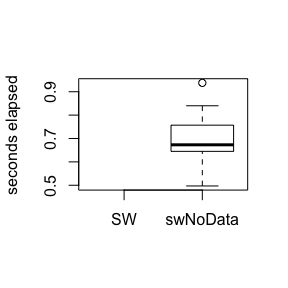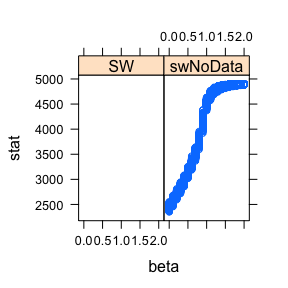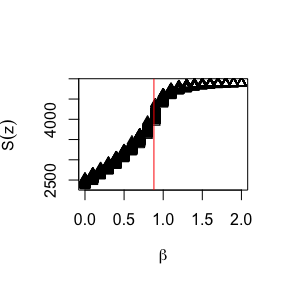# swNoData

#### 2019-01-04

Gibbs sampling was originally designed by Geman and Geman (1984) for drawing updates from the Gibbs distribution, hence the name. However, single-site Gibbs sampling exhibits poor mixing due to the posterior correlation between the pixel labels. Thus it is very slow to converge when the correlation (controlled by the inverse temperature $$\beta$$) is high.

The algorithm of Swendsen and Wang (1987) addresses this problem by forming clusters of neighbouring pixels, then updating all of the labels within a cluster to the same value. When simulating from the prior, such as a Potts model without an external field, this algorithm is very efficient.

The SW function in the PottsUtils package is implemented in a combination of R and C. The swNoData function in bayesImageS is implemented using RcppArmadillo, which gives it a speed advantage. It is worth noting that the intention of bayesImageS is not to replace PottsUtils. Rather, an efficient Swendsen-Wang algorithm is used as a building block for implementations of ABC (Grelaud et al. 2009), path sampling (Gelman and Meng 1998), and the exchange algorithm (Murray, Ghahramani, and MacKay 2006). These other algorithms will be covered in future posts.

There are two things that we want to keep track of in this simulation study: the speed of the algorithm and the distribution of the summary statistic. We will be using system.time(..) to measure both CPU and elapsed (wall clock) time taken for the same number of iterations, for a range of inverse temperatures:

beta <- seq(0,2,by=0.1)
tmMx.PU <- tmMx.bIS <- matrix(nrow=length(beta),ncol=2)
rownames(tmMx.PU) <- rownames(tmMx.bIS) <- beta
colnames(tmMx.PU) <- colnames(tmMx.bIS) <- c("user","elapsed")

We will discard the first 100 iterations as burn-in and keep the remaining 500.

iter <- 600
burn <- 100
samp.PU <- samp.bIS <- matrix(nrow=length(beta),ncol=iter-burn)

The distribution of pixel labels can be summarised by the sufficient statistic of the Potts model:

$S(z) = \sum_{i \sim \ell \in \mathscr{N}} \delta(z_i, z_\ell)$

where $$i \sim \ell \in \mathscr{N}$$ are all of the pairs of neighbours in the lattice (ie. the cliques) and $$\delta(u,v)$$ is 1 if $$u = v$$ and 0 otherwise (the Kronecker delta function). swNoData returns this automatically, but with SW we will need to use the function sufficientStat to calculate the sufficient statistic for the labels.

library(bayesImageS)

mask <- matrix(1,50,50)
neigh <- getNeighbors(mask, c(2,2,0,0))
block <- getBlocks(mask, 2)
edges <- getEdges(mask, c(2,2,0,0))

n <- sum(mask)
k <- 2
bcrit <- log(1 + sqrt(k))
maxSS <- nrow(edges)

for (i in 1:length(beta)) {
if (requireNamespace("PottsUtils", quietly = TRUE)) {
tm <- system.time(result <- PottsUtils::SW(iter,n,k,edges,beta=beta[i]))
tmMx.PU[i,"user"] <- tm["user.self"]
tmMx.PU[i,"elapsed"] <- tm["elapsed"]
res <- sufficientStat(result, neigh, block, k)
samp.PU[i,] <- res$sum[(burn+1):iter] print(paste("PottsUtils::SW",beta[i],tm["elapsed"],median(samp.PU[i,]))) } else { print("PottsUtils::SW unavailable on this platform.") } # bayesImageS tm <- system.time(result <- swNoData(beta[i],k,neigh,block,iter)) tmMx.bIS[i,"user"] <- tm["user.self"] tmMx.bIS[i,"elapsed"] <- tm["elapsed"] samp.bIS[i,] <- result$sum[(burn+1):iter]
print(paste("bayesImageS::swNoData",beta[i],tm["elapsed"],median(samp.bIS[i,])))
}
##  "PottsUtils::SW unavailable on this platform."
##  "bayesImageS::swNoData 0 0.503999999999998 2446"
##  "PottsUtils::SW unavailable on this platform."
##  "bayesImageS::swNoData 0.1 0.663000000000004 2571"
##  "PottsUtils::SW unavailable on this platform."
##  "bayesImageS::swNoData 0.2 0.838999999999999 2699.5"
##  "PottsUtils::SW unavailable on this platform."
##  "bayesImageS::swNoData 0.3 0.769000000000005 2834"
##  "PottsUtils::SW unavailable on this platform."
##  "bayesImageS::swNoData 0.4 0.938000000000002 2971"
##  "PottsUtils::SW unavailable on this platform."
##  "bayesImageS::swNoData 0.5 0.839999999999996 3137"
##  "PottsUtils::SW unavailable on this platform."
##  "bayesImageS::swNoData 0.6 0.692 3309.5"
##  "PottsUtils::SW unavailable on this platform."
##  "bayesImageS::swNoData 0.7 0.661000000000001 3519"
##  "PottsUtils::SW unavailable on this platform."
##  "bayesImageS::swNoData 0.8 0.624000000000002 3783"
##  "PottsUtils::SW unavailable on this platform."
##  "bayesImageS::swNoData 0.9 0.789999999999999 4183.5"
##  "PottsUtils::SW unavailable on this platform."
##  "bayesImageS::swNoData 1 0.671000000000006 4527"
##  "PottsUtils::SW unavailable on this platform."
##  "bayesImageS::swNoData 1.1 0.64500000000001 4676"
##  "PottsUtils::SW unavailable on this platform."
##  "bayesImageS::swNoData 1.2 0.757000000000005 4762"
##  "PottsUtils::SW unavailable on this platform."
##  "bayesImageS::swNoData 1.3 0.61999999999999 4813"
##  "PottsUtils::SW unavailable on this platform."
##  "bayesImageS::swNoData 1.4 0.673000000000002 4845"
##  "PottsUtils::SW unavailable on this platform."
##  "bayesImageS::swNoData 1.5 0.697000000000003 4863"
##  "PottsUtils::SW unavailable on this platform."
##  "bayesImageS::swNoData 1.6 0.661999999999992 4876"
##  "PottsUtils::SW unavailable on this platform."
##  "bayesImageS::swNoData 1.7 0.718999999999994 4883"
##  "PottsUtils::SW unavailable on this platform."
##  "bayesImageS::swNoData 1.8 0.521000000000001 4889"
##  "PottsUtils::SW unavailable on this platform."
##  "bayesImageS::swNoData 1.9 0.497 4893"
##  "PottsUtils::SW unavailable on this platform."
##  "bayesImageS::swNoData 2 0.711000000000013 4896"

Here is the comparison of elapsed times between the two algorithms (in seconds):

summary(tmMx.PU)
##    user         elapsed
##  Mode:logical   Mode:logical
##  NA's:21        NA's:21
summary(tmMx.bIS)
##       user          elapsed
##  Min.   :0.997   Min.   :0.4970
##  1st Qu.:1.128   1st Qu.:0.6450
##  Median :1.266   Median :0.6730
##  Mean   :1.285   Mean   :0.6901
##  3rd Qu.:1.365   3rd Qu.:0.7570
##  Max.   :1.753   Max.   :0.9380
boxplot(tmMx.PU[,"elapsed"],tmMx.bIS[,"elapsed"],ylab="seconds elapsed",names=c("SW","swNoData"))On average, swNoData using RcppArmadillo (Eddelbuettel and Sanderson 2014) is seven times faster than SW.

s_z <- c(samp.PU,samp.bIS)
s_x <- rep(beta,times=iter-burn)
s_a <- rep(1:2,each=length(beta)*(iter-burn))
s.frame <- data.frame(s_z,c(s_x,s_x),s_a)
names(s.frame) <- c("stat","beta","alg")
s.frame$alg <- factor(s_a,labels=c("SW","swNoData")) if (requireNamespace("lattice", quietly = TRUE)) { lattice::xyplot(stat ~ beta | alg, data=s.frame) }plot(c(s_x,s_x),s_z,pch=s_a,xlab=expression(beta),ylab=expression(S(z))) abline(v=bcrit,col="red")The overlap between the two distributions is almost complete, although it is a bit tricky to verify that statistically. The relationship between $$beta$$ and $$S(z)$$ is nonlinear and heteroskedastic. rowMeans(samp.bIS) - rowMeans(samp.PU) ##  NA NA NA NA NA NA NA NA NA NA NA NA NA NA NA NA NA NA NA NA NA apply(samp.PU, 1, sd) ##  NA NA NA NA NA NA NA NA NA NA NA NA NA NA NA NA NA NA NA NA NA apply(samp.bIS, 1, sd) ##  34.159419 34.260593 34.919729 35.856902 38.198227 41.902192 42.360515 ##  47.940617 54.571909 74.725912 44.237831 33.328516 24.102557 20.061483 ##  15.361047 12.389091 10.179723 8.006049 6.686276 5.617966 4.259688 s.frame$beta <- factor(c(s_x,s_x))
if (requireNamespace("PottsUtils", quietly = TRUE)) {
s.fit <- aov(stat ~ alg + beta, data=s.frame)
summary(s.fit)
TukeyHSD(s.fit,which="alg")
}